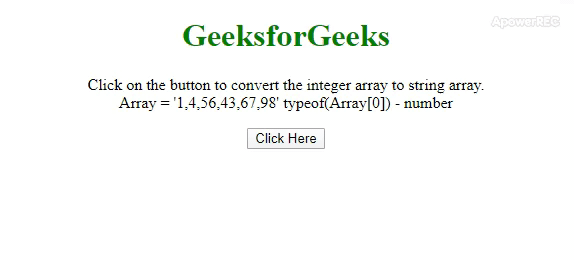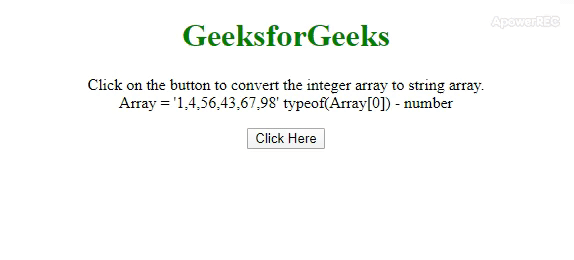# How to convert Integer array to String array using JavaScript ?

The task is to convert an integer array to the string array. Here a few of the most used techniques discussed with the help of JavaScript. In the first approach we are going to use the .toString() method with the .map() method and in the seconds approach we will use .join() method with .split() method.

Approach 1: In this approach we use .toString() method on every element of the array with the help of .map() method.

• Example: This example uses the approach discussed above.

 ` ` `<``html``> ` ` `  `<``head``> ` `    ``<``title``> ` `        ``How to Convert Integer array to ` `        ``String array using JavaScript ? ` `    `` ` `     `  `    ``<``style``> ` `        ``body { ` `            ``text-align: center; ` `        ``} ` `        ``h1 { ` `            ``color: green; ` `        ``} ` `        ``#geeks{ ` `            ``font-weight: bold; ` `        ``} ` `    `` ` ` ` ` `  `<``body``> ` `    ``<``h1``>GeeksforGeeks ` `     `  `    ``<``p` `id``=``"GFG"``> ` `     `  `    ``<``button` `onclick``=``"gfg_Run()"``> ` `        ``Click Here ` `    `` ` `     `  `    ``<``p` `id``=``"geeks"``> ` `     `  `    ``<``script``> ` `        ``var el_up = document.getElementById("GFG"); ` `        ``var el_down = document.getElementById("geeks"); ` `        ``var arr = [1, 4, 56, 43, 67, 98]; ` `         `  `        ``el_up.innerHTML = "Click on the button to " ` `                ``+ "convert the integer array" +  ` `                ``" to string array.<``br``>Array = '" ` `                ``+ arr + "' typeof(Array) - " ` `                ``+ typeof(arr); ` `         `  `        ``function gfg_Run() { ` `            ``var gfg = arr.map(function(e){ ` `                ``return e.toString() ` `            ``}); ` `                 `  `            ``el_down.innerHTML = "Array - " + gfg +  ` `                ``" typeof(Array) - " + typeof(gfg);  ` `        ``}  ` `    ``  ` ` ` ` `  ` `

• Output:Approach 2: In this approach we use .join() method which joins the array and returns it as a string. Then .split() method which splits the string on “, ” returned by join() method.

• Example: This example uses the approach discussed above.

 ` ` `<``html``> ` ` `  `<``head``> ` `    ``<``title``> ` `        ``How to Convert Integer array to ` `        ``String array using JavaScript ? ` `    `` ` ` `  `    ``<``style``> ` `        ``body { ` `            ``text-align: center; ` `        ``} ` `        ``h1 { ` `            ``color: green; ` `        ``} ` `        ``#geeks{ ` `            ``font-weight: bold; ` `        ``} ` `    `` ` ` ` ` `  `<``body``> ` `    ``<``h1``>GeeksforGeeks ` `     `  `    ``<``p` `id``=``"GFG"``> ` `     `  `    ``<``button` `onclick``=``"gfg_Run()"``> ` `        ``Click Here ` `    `` ` `     `  `    ``<``p` `id``=``"geeks"``> ` `     `  `    ``<``script``> ` `        ``var el_up = document.getElementById("GFG"); ` `        ``var el_down = document.getElementById("geeks"); ` `        ``var arr = [1, 4, 56, 43, 67, 98]; ` `         `  `        ``el_up.innerHTML = "Click on the button to " ` `                ``+ "convert the integer array" + ` `                ``" to string array.<``br``>Array = '"  ` `                ``+ arr + "' typeof(Array) - " ` `                ``+ typeof(arr); ` ` `  `        ``function gfg_Run() { ` `            ``var gfg = arr.join().split(', '); ` `             `  `            ``el_down.innerHTML = "Array - " + gfg +  ` `            ``" typeof(Array) - " + typeof(gfg); ` `        ``} ` `    `` ` ` ` ` `  ` `

• Output:My Personal Notes arrow_drop_upCheck out this Author's contributed articles.

If you like GeeksforGeeks and would like to contribute, you can also write an article using contribute.geeksforgeeks.org or mail your article to contribute@geeksforgeeks.org. See your article appearing on the GeeksforGeeks main page and help other Geeks.

Please Improve this article if you find anything incorrect by clicking on the "Improve Article" button below.